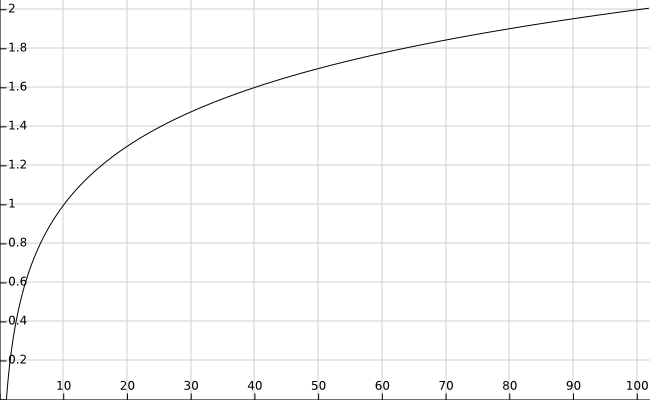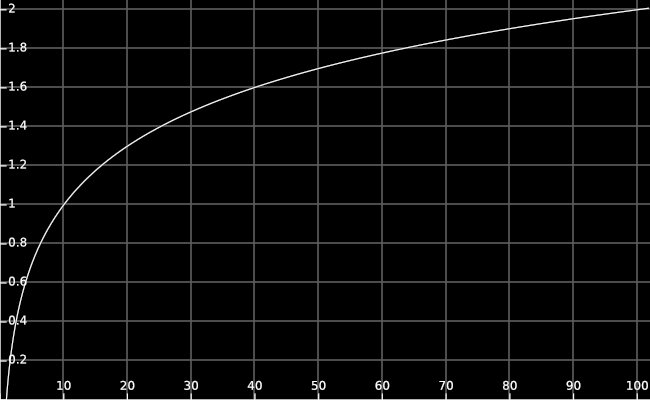# What is a logarithm?

Logarithms are a group of functions that map numbers to numbers. There is a logarithm function for every positive number $$b$$ except 1, and the logarithm function for $$b$$ (called “the logarithm base $$b$$” or just “log base $$b$$”) takes an input $$x$$ and counts how many times you have to multiply $$1$$ by $$b$$ to get $$x$$.

For example, the log base 10 of 1000 is 3, because you have to multiply 1 by 10 three times to get 1000, and the log base 2 of 16 is 4, because you have to multiply 1 by 2 four times to get 16. The log base $$b$$ of $$x$$ is written $$\log_b(x).$$

The logarithm function grows slowly. For example, $$\log_{10}(x)$$ is less than 1 until $$x$$ reaches 10, and it’s less than 2 until $$x$$ reaches 100, and it’s less than 6 until $$x$$ reaches 1,000,000 and so on. You’ve got to put a lot of oomph (specifically, a whole factor of 10) into the input in order to make the output go up by 1. Here’s a graph of $$\log_{10}(x)$$ inching its way towards a measly 2 as $$x$$ makes it all the way out to 100:Make this graph interactive.

Logarithms may grow slowly, but they never stop growing: For every number $$n$$, no matter how large, there is an input $$x$$ such that $$\log_{10}(x) > n.$$

## Examples

1. $$\log_{10}(10000) = 4,$$ because you have to multiply 1 by 10 four times to get ten thousand: $$1 \cdot 10 \cdot 10 \cdot 10 \cdot 10 = 10000.$$

2. $$\log_2(8) = 3,$$ because $$1 \cdot 2 \cdot 2 \cdot 2 = 8.$$

3. $$\log_3(9) = 2,$$ because $$1 \cdot 3 \cdot 3 = 9.$$

4. $$\log_{b}(1) = 0$$ for any $$b$$, because no matter what $$b$$ is, you don’t need to multiply 1 by $$b$$ any times to get 1.

5. $$\log_{b}(b) = 1$$ for any $$b$$, because no matter what $$b$$ is, if you multiply 1 by $$b$$ once, you get $$b.$$

6. $$\log_{1.5}(3.375) = 3,$$ because $$1 \cdot 1.5 \cdot 1.5 \cdot 1.5 = 3.375.$$

Question: What’s $$\log_3(27)$$?

Answer: 3, because $$1 \cdot 3 \cdot 3 \cdot 3 = 27$$.

Question: What’s $$\log_4(16)$$?

Answer: 2, because $$1 \cdot 4 \cdot 4 = 16$$.

Question: What’s $$\log_{10}(\text{1,000,000})$$?

Answer: 6, because $$1 \cdot 10 \cdot 10 \cdot 10 \cdot 10 \cdot 10 \cdot 10 = 1,000,000$$.

All of the examples above are cases where the answer is a whole number, because $$x$$ is a power of $$b.$$ Logarithms also give answers in cases where the answer is not a whole number. For example, $$\log_{10}(500) \approx 2.7$$.

What’s that supposed to mean? That if you multiply 1 by 10 about 2.7 times, you get 500?

Yes, precisely! If you multiply 1 by ten once, and multiply the result by ten another time, and then multiply that by ten 0.7 times, the result is roughly 500. What does it mean to multiply a number by ten 0.7 times? Well, is there a number $$x$$ such that if we multiply 1 by $$x$$ ten times, it’s the same as multiplying 1 by 10 seven times? Yes! That number is roughly 5:

$$\underbrace{5 \cdot 5 \cdot \ldots 5}_\text{10 times} \approx \underbrace{10 \cdot 10 \cdot \ldots 10}_\text{7 times}$$

Multiplying by 5 (ten times) is about the same as multiplying by 10 (seven times), so multiplying by five (once) is about the same as multiplying by 10 (seven-tenths of a time). Thus, multiplying 1 by ten 2.7 times means multiplying it by 10 twice, then multiplying it by approximately 5, for a result of approximately 500.

This is why $$\log_{10}(500) \approx 2.7$$: that’s how many times you need to multiply 1 by 10 to get 500.

If that answer isn’t satisfactory yet, don’t worry: Throughout the tutorial, we’ll explore both (a) what this means, and (b) why this is the “right way” to multiply a number by $$b$$ fractionally-many times.

You might be saying to yourself, “Ok, I can see how 500 is kinda sorta one times ten 2.7 times, but how do you figure that out? As far as I can tell, you pulled that “$5^{10}$ is approximately $$10^7$$” thing out of thin air. Where did that come from? How is the logarithm actually calculated?”

The annoying answer is that I calculated $$\log_{10}(500)$$ by typing “log10(500)” into Wolfram Alpha. In more seriousness, in the coming posts, you’ll learn how to find things like $$\log_{10}(500)$$ on your own.

That said, the outputs of logarithms are generally difficult to find, but useful once you’ve found them. Historically, scientists and engineers would pay money for giant tables of pre-computed logarithm values, because those sped up their practical calculations considerably. Why are the outputs of logarithm functions so convenient to use? That question will be answered later in this tutorial.

You might also be saying to yourself, “ok, I buy that there’s a way to see 500 as ‘1 times 10, 2.7 times,’ but what does that mean? What’s the point of looking at 500 that way?”

It’s hard to give a short answer to that question, but only because there are so many good answers. One answer goes something like, “if you think of 500 as 2.7 tens, and you think of 8000 as 3.9 tens, then you can multiply the numbers on the left by adding the representations on the right”. For example, $$500 \cdot 8000$$ is approximately $$2.7 + 3.9 = 6.6$$ tens.

But that’s getting ahead of ourselves a bit. The most intuitive interpretation to start with is probably this one: Logarithms are measuring how “long” a number is, in terms of how many digits it takes to write that number down, for a generalized notion of “length.”

It may seem that numbers always take a whole number of digits to write down: 139 and 931 are both written using three digits (‘9’, ‘3’, and ‘1’). However, as we will see, there’s a sense in which 139 is barely using its third digit, while 931 is using all three of its digits to almost their full extent. Logarithms quantify this intuition, and the precise answer that they give reveals some interesting facts about how many digits it costs to write a given number down.

Parents:

• Seven tenths?

• This paragraph is good—clearer than some of the other places where you tried to introduced this idea.